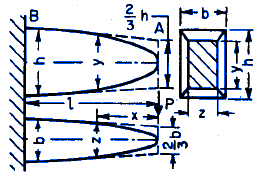Cubic Parabola Shape Fixed End Single Concentrated Force Applied Beam Deflection

Beam Cubic Parabola Shape Deflection Equation Calculator with Variable Shape Fixed End Single Concentrated Force Applied

• Cross Section: Rectangle: width z variable, depth y constant
• Elevation: Cubic Parabola
• Plan: Cubic ParabolaALL calculators require a Premium Membership

Equationsk (Constant) = z / y

I2 = b3 d / 30

c = b / 2

Ss = I2 / cb1 = k h

Deflection at A:

f = ( 6 P / b1 E ) ( l / h )3

Where:

 E = Modulus of Elasticity psi (N/mm2) I = Moment of Inertia in4 (mm4) P = Force lbf (N) f = Deflection inches (mm) Ss= Section Modulus in3 (mm3) a, b, c, d, x, l = Some distance as indicated inches (mm)

References:

• Civil Engineering Formulas, Tyler G. Hicks, PE International Engineering Associates© Copyright 2000 - 2019, by Engineers Edge, LLC www.engineersedge.comMembership Register | LoginHomeEngineering Book StoreEngineering ForumExcel App. DownloadsOnline Books & ManualsEngineering NewsEngineering VideosEngineering CalculatorsEngineering ToolboxGD&T Training Geometric Dimensioning TolerancingDFM DFA TrainingTraining Online EngineeringAdvertising CenterCopyright Notice